# Error calibration

The first step to handling errors is to quantify them. Make sure that your data's “dilution of precision” (DOP) and error columns import correctly into `ctmm`. Some tracking device manufacturers provide pre-calibrated errors. These coati were tracked with e-obs GPS collars that features calibrated errors for the location, imported by `ctmm` into the `VAR.xy` column, which represents the `x` and `y` error variances.

``````library(ctmm)
data('coati')
names(coati[]) # imported column names
``````
``````##   "timestamp" "longitude" "latitude"  "t"         "HDOP"
##   "VAR.xy"    "z"         "VDOP"      "speed"     "heading"
##  "SDOP"      "VAR.v"     "x"         "y"         "vx"
##  "vy"
``````
``````plot(coati[],col=rainbow(2),error=2,trans=0.4) # coati plot with 95% error discs
``````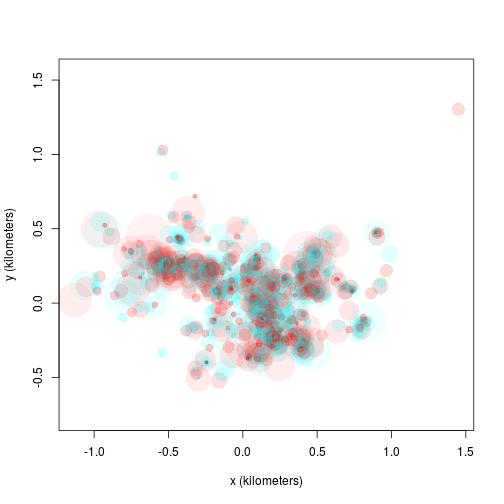Most GPS devices will only have an `HDOP` column, which is only proportional to the `x` and `y` error standard deviations. The proportionality constant between the HDOP and error standard deviation is known as the user equivalent range error (UERE). The UERE should be estimated from calibration data if not provided by the manufacturer. Without the option of pre-calibrated errors or calibration data, the UERE must be fit simultaneously with the movement model, which is not as reliable. We will cover simultaneous fitting at the end of this vignette.

In the following wood turtle dataset, we have some calibration data and a turtle track. Note that the calibration data must be collected from the same model device as the tracking data.

``````data(turtle)
names(turtle[]) # data are not yet calibrated
``````
``````##  "t"    "HDOP" "x"    "y"
``````
``````names(turtle) # some calibration data and some turtle data
``````
``````##  "Calibration.1" "Calibration.2" "Turtle.1"      "Turtle.2"
``````
``````plot(turtle[1:2],col=rainbow(2)) # calibration data only
``````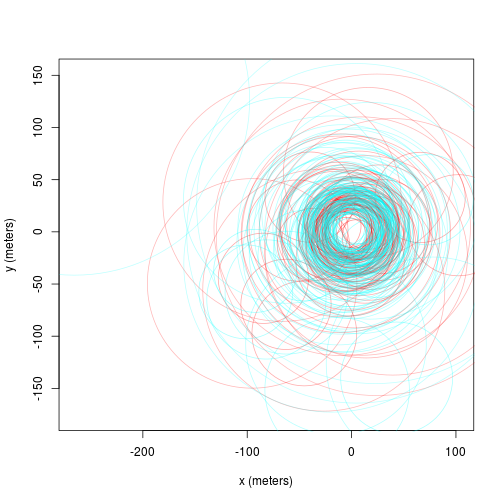The `uere` command is used to estimate the UERE parameter from calibration data. Do not use this command on animal tracking data.

``````UERE <- uere.fit(turtle[1:2]) # only using calibration data
summary(UERE)
``````
``````## , , horizontal
##
##          low       ML     high
## all 9.940412 10.62878 11.31654
``````

For GPS data, the UERE will typically be 10-15 meters. The UERE can be assigned to a dataset with the `uere()<-` command.

``````uere(turtle) <- UERE
names(turtle[]) # now the data are calibrated
``````
``````##  "t"      "HDOP"   "x"      "y"      "VAR.xy"
``````
``````plot(turtle[],error=2) # turtle plot with 95% error discs
``````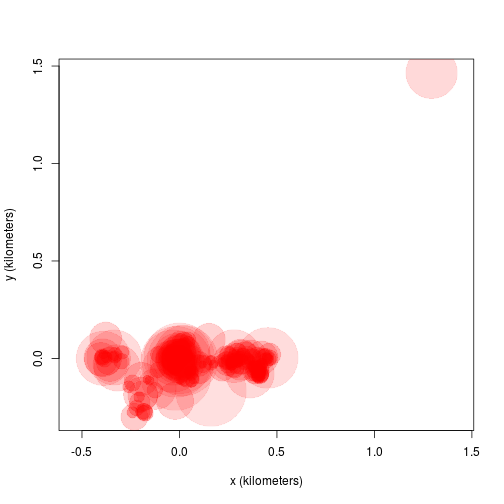# Outlier detection

Now we come to the task of identifying outliers. The `outlie` function uses error information to estimate straight-line speeds between sampled times and distances from the bulk of the data.

``````outlie(turtle[]) -> OUT
``````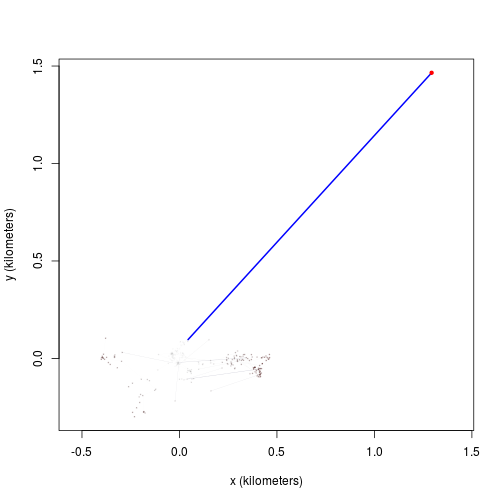High-speed segments are colored in blue, while distant locations are colored in red. More emphasis is placed on the more extreme locations in the `outlie` plot. Visually we can see at least one outlier in the wood turtle data. The output of `outlie` also contains the error-informed speed and distance estimates (in SI units) used to generate the plot.

``````plot(OUT)
``````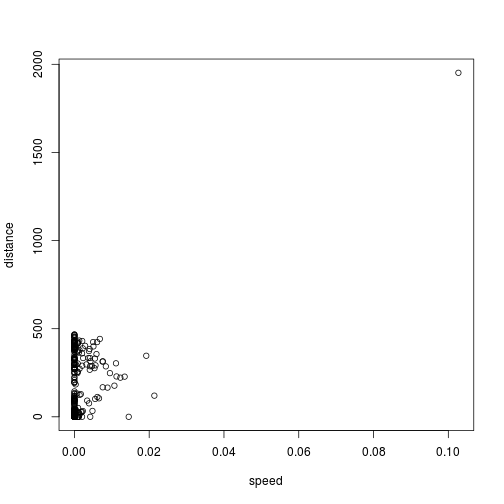A sustained speed of 0.1 m/s is not biologically implausible for a wood turtle, but this location is highly suspicious, both in terms of speed and lack of proximity. After removing the outlier, we can check the data again.

``````BAD <- which.max(OUT\$speed)
outlie(turtle[]) -> OUT
``````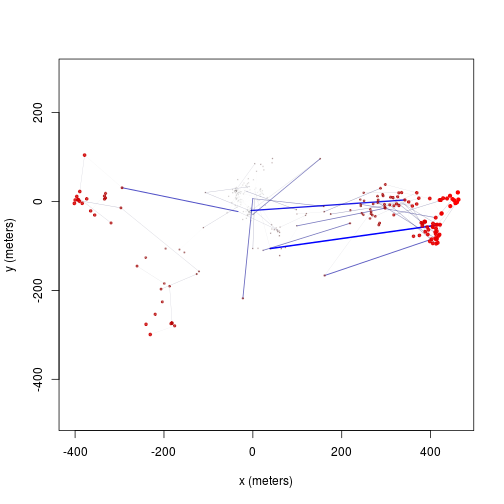Datasets may have multiple outliers. In pathological situations, there may be no clear separation between the normative data and the outliers. This necessitates a better error model, either by improving inadequate (or absent) HDOP estimates or by employing a heavier tailed error distribution (not yet supported).

# Variograms and model selection

## Variograms

If we were working with ARGOS data or high resolution GPS data on a small animal, then we can get a “nugget” effect in the variogram that looks like an initial discontinuity at short time lags.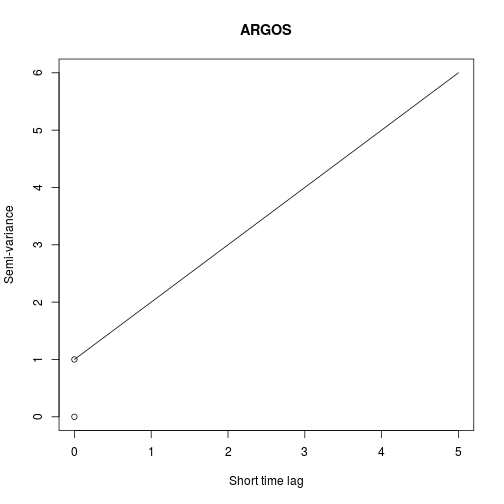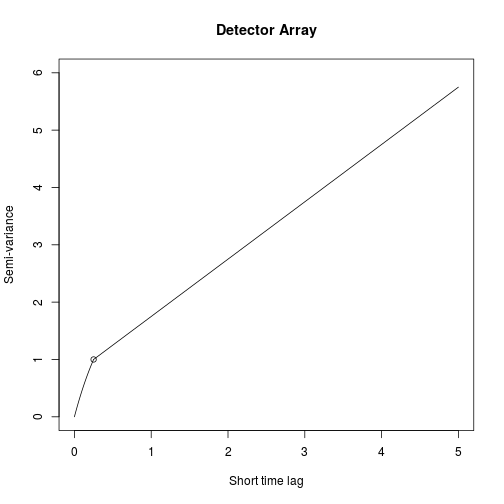The height of this initial discontinuity corresponds to the variance of uncorrelated location errors. The second plot is the kind of initial discontinuity one has with detector array data. The end of the (slope) discontinuity is highlighted with a circle. This discontinuity is smooth because the movement and detection are correlated. The height of this initial discontinuity is also (at least roughly) the variance of the location errors.

## Model fitting

Because of some convenient mathematical relations, fitting with telemetry errors involves numerically fitting 1-4 more parameters and is, therefore, slower and less reliable at converging on the MLE than fitting without telemetry error. Therefore, by default, telemetry error is not turned on in `ctmm` models (`error=FALSE`). Furthermore, in cases with an error model, `ctmm.select()` is safer to use than direct applicaiton of `ctmm.fit()`, because `ctmm.select()` will start with the simplest “compatible” model and then consider further model features that require numerical optimization in stepwise fashion.

### Fitting with calibrated data

For calibrated errors, we have to set `error=TRUE` in the model guess to fit with telemetry error accounted for.

``````# automated guestimates with circular covariance and calibrated errors
GUESS <- ctmm.guess(turtle[],CTMM=ctmm(error=TRUE),interactive=FALSE)
# the beta optimizer is more reliable than the default optimizer
# control <- list(method='pNewton',cores=-1) # will use all but one cores
control <- list(method='pNewton',cores=2) # CRAN policy limits us to 2 cores
# stepwise fitting
FIT <- ctmm.select(turtle[],GUESS,control=control,trace=TRUE)
``````
``````## * Fitting model OUF isotropic error
``````
``````## * Fitting models OUF anisotropic error
``````
``````## * Fitting models OU anisotropic error
``````
``````summary(FIT)
``````
``````## \$DOF
##     mean     area    speed
## 12.59923 24.41651  0.00000
##
## \$CI
##                           low        ML     high
## area (hectares)     12.192644 18.949154 27.17140
## tau position (days)  3.693129  6.319046 10.81206
``````

`trace=TRUE` allows us to see the models considered. `verbose=TRUE` would save each model.

### Fitting with uncalibrated data

Fitting with unknown errors is a method of last resort. We have to provide an initial guess for `error`, which will be the device's UERE. `error=10` is usually a good guess for GPS data. Let's consider the turtle data again, but without calibrated UERE.

``````# delete UERE information
uere(turtle[]) <- NULL
``````

The only difference is that you have to provide an initial guess for the UERE. Otherwise, the steps are the same.

``````# cheat and use previous fit as initial guess
GUESS\$error <- 10 # 10 meter error guess
# fit parameter estimates
FIT <- ctmm.select(turtle[],GUESS,control=control)
summary(FIT)
``````
``````## \$DOF
##     mean     area    speed
## 13.46151 25.98177  0.00000
##
## \$CI
##                           low        ML      high
## area (hectares)     12.604531 19.298800 27.396178
## tau position (days)  3.467312  5.882937  9.981492
## error (meters)       0.000000  9.317617 63.303345
``````

Here, fitting the UERE simultaneously with the movement model produced a consistent result. This will not always be the case.